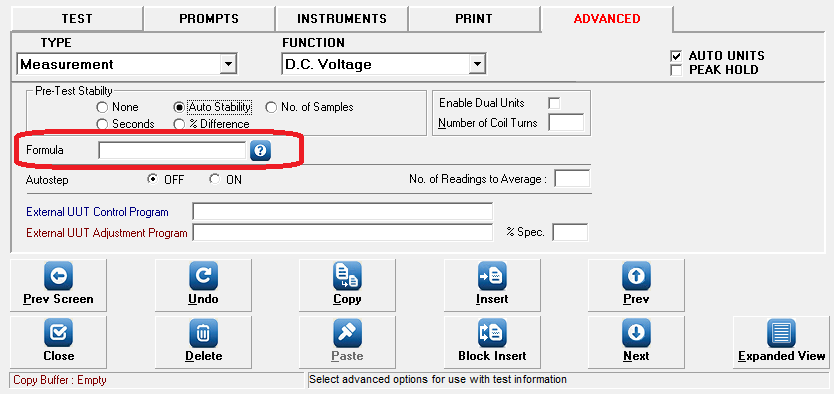Modified on Thu, 18 Aug 2016 at 10:31 AM

ProEdit / ProCal feature the ability to manipulate results through use of a Formula field in the ADVANCED tab of the ProEdit procedure editing screenUsing the FORMULA field results can be divided, multiplied, added or subtracted by fixed numbers, previous tests or by the values of standards in ProSet

To use the formula box, first select the test(s) that require formulas, and then enter the mathematical formula that you wish to perform, using the following naming scheme

X : X represents the current reading, obtained over the interface or entered in the entry box
N : N refers to the nominal value of the test

Tx : T refers to a test number, i.e. T3 would refer to the value obtained for Test 3

Ex : E refers to the VALUE of a standard stored in ProSet.

Examples :

X=X/E30 : Divide the obtained reading by the value of the standard stored in location 30 of ProSet (used for ohms law calculations)

N=T3 : Set the nominal value of the current test as the result of Test 3 (i.e. measuring the temperature of a water bath)

X-T1 : Subtract the value of T1 from the current reading (i.e. removing the zero of a multimeter)